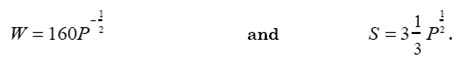### Create an Account

Home / Questions / The dominant firm model can help us understand the behavior of some cartels Let’s apply t...

# The dominant firm model can help us understand the behavior of some cartels Let’s apply this model to the OPEC oil cartel We shall use isoelastic curves to describe world demand W and noncartel

The dominant firm model can help us understand the behavior of some cartels.  Let’s apply this model to the OPEC oil cartel.  We shall use isoelastic curves to describe world demand W and noncartel (competitive) supply S.  Reasonable numbers for the price elasticities of world demand and non-cartel supply are -1/2 and 1/2, respectively.  Then, expressing W and S in millions of barrels per day (mb/d), we could writeNote that OPEC’s net demand is D = W - S.

a. Sketch the world demand curve W, the non-OPEC supply curve S, OPEC’s net demand curve D, and OPEC’s marginal revenue curve.  For purposes of approximation, assume OPEC’s production cost is zero.  Indicate OPEC’s optimal price, OPEC’s optimal production, and non-OPEC production on the diagram.  Now, show on the diagram how the various curves will shift, and how OPEC’s optimal price will change if non-OPEC supply becomes more expensive because reserves of oil start running out.

b. Calculate OPEC’s optimal (profit-maximizing) price.  (Hint: Because OPEC’s cost is zero, just write the expression for OPEC revenue and find the price that maximizes it.)

c. Suppose the oil-consuming countries were to unite and form a “buyers’ cartel” to gain monopsony power.  What can we say, and what can’t we say, about the impact this would have on price?

May 07 2020 View more View LessSubscribe To Get Solution